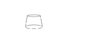# What is the volume of this tree using integral?

• -EquinoX-
Just plug the radius values in and you're done.How can a linear function be a really nasty function? You are actually integrating thearea of a disk so the function you need to integrate is quadratic- that's not "really nasty"!

#### -EquinoX-

The circumference of a tree at different heights above the ground is given in the table below.
Assume that all horizontal cross-sections of the tree are circles.
Estimate the volume of the tree. (Measurements are in inches.)

$$\begin{array}{cccccccc}\text{Height} & 0 & 20 & 40 & 60 & 80 & 100 & 120 \\ \text{Circumference} & 31 & 28 & 21 & 17 & 12 & 8 & 2\end{array}$$

I already try to do this by finding the linear function of the radius of the tree from 0-20, 20-40 and so on but this takes a long time. I have to solve this using integrals, is there any faster way to do this?

Last edited:
Hrm. So, you want to approximate the value of an integral knowing the value of the integrand at several points?

Umm use some form of numerical integration to find the area of the corss section of half the tree. Then divide this by the total height to find the average radius, use that value of r for your cylinder :)

Gib Z said:
Umm use some form of numerical integration to find the area of the corss section of half the tree. Then divide this by the total height to find the average radius, use that value of r for your cylinder :)
It's less work to estimate the volume directly, and probably more accurate too!

-EquinoX- said:
The circumference of a tree at different heights above the ground is given in the table below.
Assume that all horizontal cross-sections of the tree are circles.
Estimate the volume of the tree. (Measurements are in inches.)

$$\begin{array}{cccccccc}\text{Height} & 0 & 20 & 40 & 60 & 80 & 100 & 120 \\ \text{Circumference} & 31 & 28 & 21 & 17 & 12 & 8 & 2\end{array}$$

I already try to do this by finding the linear function of the radius of the tree from 0-20, 20-40 and so on but this takes a long time. I have to solve this using integrals, is there any faster way to do this?

Like Hurkyl says, why not just work out the volume of all the shapes from bottom to top?

https://www.physicsforums.com/attachment.php?attachmentid=9189&stc=1&d=1171719342

#### Attachments

•shape.JPG
2.1 KB · Views: 1,725
so what you mean here I just find the linear function from 0-120 and then just take the integral of it from 0 to 120 phi*(the linear function)^2 dx

A "quick and dirty" way to do this would be to treat the radius as being a constant from each level up to the next, find the volume of each cylinder and add.

If you use a "piecewise linear" function to be more accurate, then you could do each one as a truncated cone.

HallsofIvy said:
A "quick and dirty" way to do this would be to treat the radius as being a constant from each level up to the next, find the volume of each cylinder and add.

If you use a "piecewise linear" function to be more accurate, then you could do each one as a truncated cone.

this is what I've been doing now, but it takes a really long time. the linear function of the radius is a really nasty function to take integral of

-EquinoX- said:
this is what I've been doing now, but it takes a really long time. the linear function of the radius is a really nasty function to take integral of
Only if you grind it through: you (should have) learned how to evaluate this integral in your geometry classes, though you might have called it "finding the volume of this shape" in that course...

(And, there is a simpler approximation than linear interpolation, though it's usually not as accurate)

And is it really a nasty integral? It sounds as if it's straightforward. Well, you only have to integrate it once anyways: you should do it with variables, and then plug in your actual numbers into the resulting formula.

Last edited:
Math

I think on this specific problem you just have to use Simpson's rule in order to approximate the integral. V=2πrh for any small part of the tree considering the radius remains constant. in order to get the approximate volume you use any of the numerical methods of integration such as Simpson's rule of trapezoid rule.

#### Attachments

thanks Bruk, that really clears up the problem and probably the shortest way to do it

-EquinoX- said:
this is what I've been doing now, but it takes a really long time. the linear function of the radius is a really nasty function to take integral of

How can a linear function be a really nasty function? You are actually integrating the area of a disk so the function you need to integrate is quadratic- that's not "really nasty"!

Actually, you could use the formula for a frustrum of a cone and not do any integration at all.